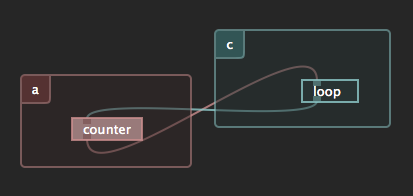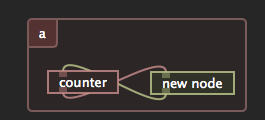# Network loop to test latency

Now that I can create and remove links between processes, I thought of a little experiment:

What happens if I create a loop ?Here’s the content of both scripts:

### counter

```inlet('bang', 'Send next number on [bang].')
pass = outlet('pass', 'Increasing numbers [float]')

function inlet.bang(i)
i = i + 1
print(i)
pass(i)
end

-- initiates the loop on script save
pass(0)
```

### loop

```inlet('bang', 'Send next number on [bang].')
pass = outlet('pass', 'Increasing numbers [float]')

function inlet.bang(i)
i = i + 1
pass(i)
end
```

### results

I stated the script and I let it run for 30s. The counter reached 241’558 in these 30 seconds which means 8’050 messages per second.

The monitor (open during the test) shows 2.4Mb/s of data being transferred.

The same loop in a single process gives this result 1’298’724 (43’290 messages per second). Note that I had to change the “counter” so that it uses “app:post” to send in order to avoid a stack overflow error (in-process messaging is the same as a function call):Updated “counter” inlet function:

```function inlet.bang(i)
i = i + 1
print(i)
app:post(function()
-- post to avoid stack overflow
count(i)
end)
end

count(0)
```